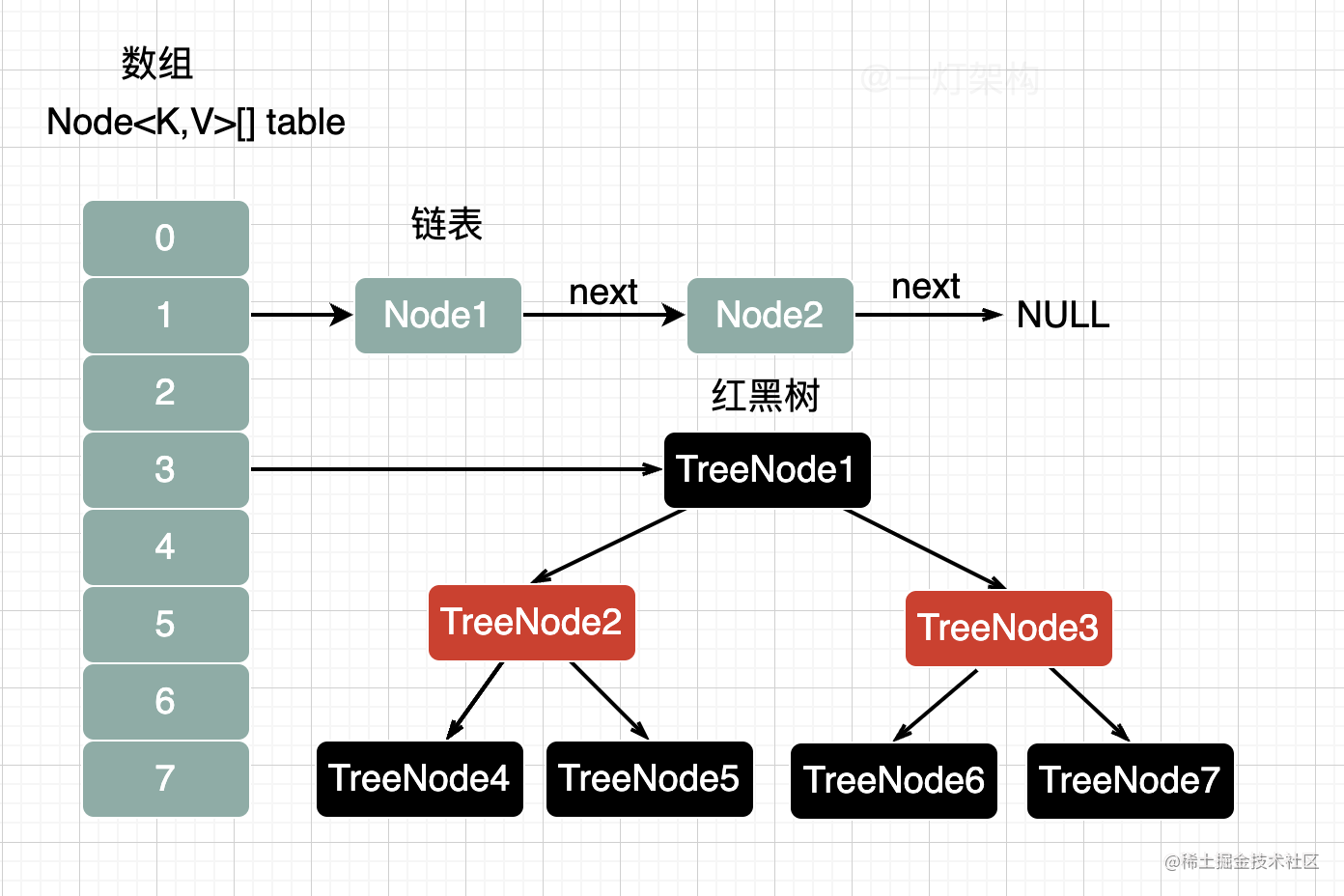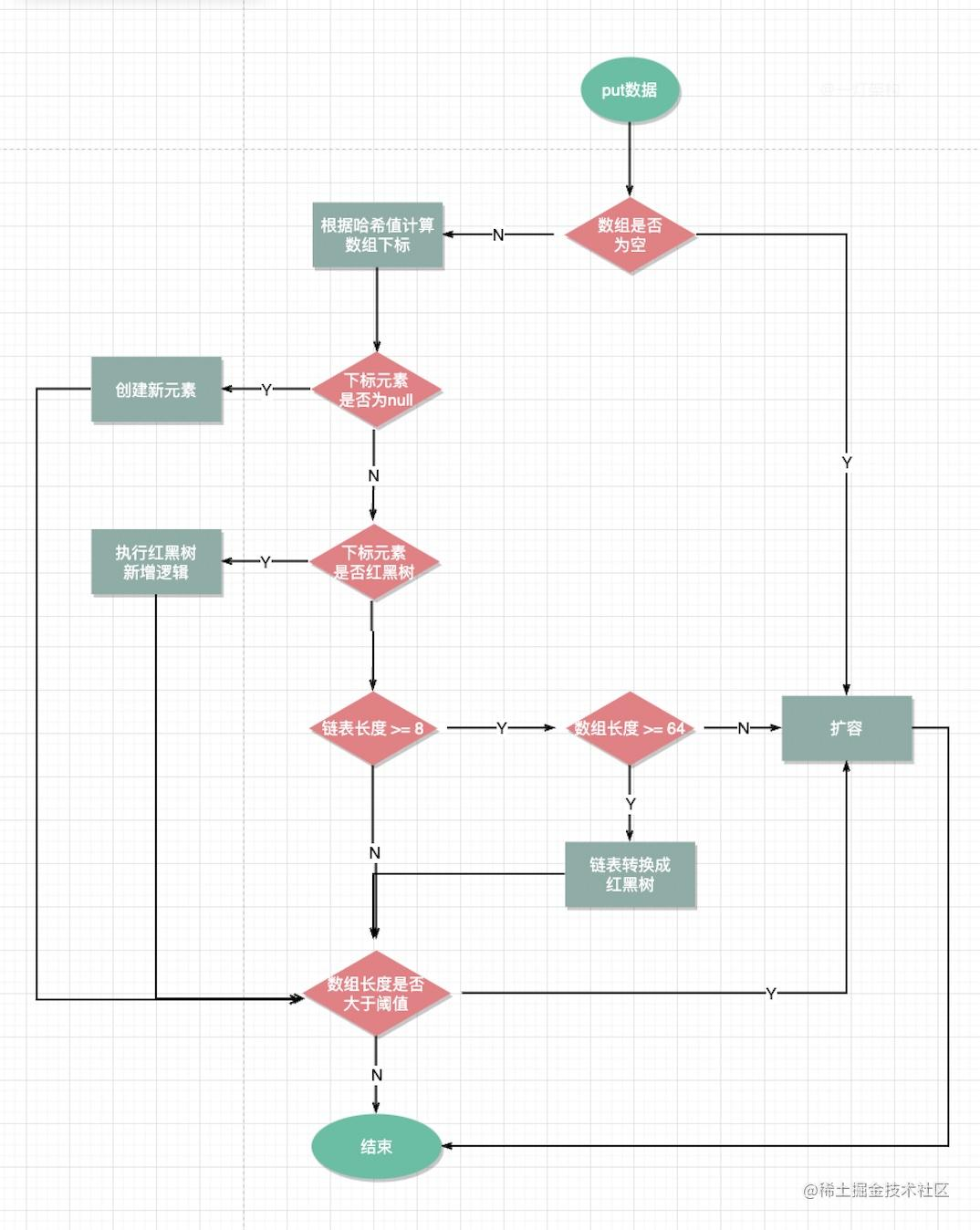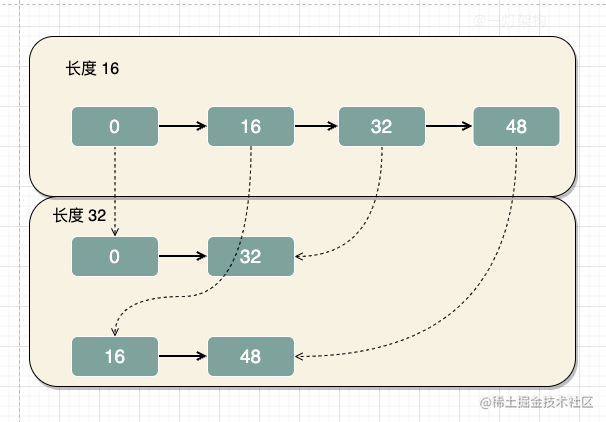# 我说HashMap初始容量是16，面试官让我回去等通知

## 1. HashMap底层数据结构？

JDK1.7采用的是数组+链表，数组可以通过下标访问，实现快速查询，链表用来解决哈希冲突。

JDK1.8采用的是数组+链表+红黑树的结构，当链表长度大于等于8，并且数组长度大于等于64时，链表才需要转换成成红黑树。## 2. HashMap的初始容量是多少？

JDK1.7的时候初始容量确实是16，但是JDK1.8的时候初始化HashMap的时候并没有指定容量大小，而是在第一次执行put数据，才初始化容量。

```java // 负载因子大小 final float loadFactor;

// 默认负载因子大小 static final float DEFAULT_LOAD_FACTOR = 0.75f;

## 3. HashMap的put方法流程？

1. 计算key的哈希值
2. 判断数组是否为空，如果为空，就执行扩容，初始化数据大小。
3. 如果数组不为空，根据哈希值找到数组所在下标
4. 判断下标元素是否为null，如果为null就创建新元素
5. 如果下标元素不为null，就判断是否是红黑树类型，如果是，则执行红黑树的新增逻辑
6. 如果不是红黑树，说明是链表，就追加到链表末尾
7. 如果判断链表长度是否大于等于8，数组长度是否大于等于64，如果不是就执行扩容逻辑
8. 如果是，则需要把链表转换成红黑树
9. 最后判断新增元素后，判断元素个数是否大于阈值（16*0.75=12），如果是则执行扩容逻辑，结束。```java // put方法入口 public V put(K key, V value) { // 计算哈希值 return putVal(hash(key), key, value, false, true); }

final V putVal(int hash, K key, V value, boolean onlyIfAbsent, boolean evict) { Node[] tab; Node p; int n, i; // 判断数组是否为空，为空的话，则进行扩容初始化 if ((tab = table) == null || (n = tab.length) == 0) n = (tab = resize()).length; // 计算数组下标位置，判断下标位置元素是否为null if ((p = tab[i = (n - 1) & hash]) == null) tab[i] = newNode(hash, key, value, null); // 数组中已经存在元素（处理哈希冲突） else { Node e; K k; // 判断元素值是否一样，如果一样则替换旧值 if (p.hash == hash && ((k = p.key) == key || (key != null && key.equals(k)))) e = p; // 判断元素类型是否是红黑树 else if (p instanceof TreeNode) // 执行红黑树新增逻辑 e = ((TreeNode)p).putTreeVal(this, tab, hash, key, value); // 不是红黑树类型则说明是链表 else { // 遍历链表 for (int binCount = 0; ; ++binCount) { // 到达链表的尾部 if ((e = p.next) == null) { // 在尾部插入新结点 p.next = newNode(hash, key, value, null); // 链表结点数量达到阈值(默认为 8 )，执行 treeifyBin 方法 // 这个方法会根据 HashMap 数组来决定是否转换为红黑树。 // 只有当数组长度大于或者等于 64 的情况下，才会执行转换红黑树操作，以减少搜索时间。否则，就是只是对数组扩容。 if (binCount >= TREEIFY_THRESHOLD - 1) treeifyBin(tab, hash); break; } // 判断链表中结点的key值与插入的元素的key值是否相等 if (e.hash == hash && ((k = e.key) == key || (key != null && key.equals(k)))) // 相等，跳出循环 break; // 用于遍历桶中的链表，与前面的e = p.next组合，可以遍历链表 p = e; } } // 表示在数组中找到key值、哈希值与插入元素相等的结点 if (e != null) { // 记录e的value V oldValue = e.value; // onlyIfAbsent为false或者旧值为null if (!onlyIfAbsent || oldValue == null) //用新值替换旧值 e.value = value; // 访问后回调 afterNodeAccess(e); // 返回旧值 return oldValue; } } // 记录修改次数 ++modCount; // 元素个数大于阈值则扩容 if (++size > threshold) resize(); // 插入后回调 afterNodeInsertion(evict); return null; } ```

## 4. HashMap容量大小为什么要设置成2的倍数？

```java int index = hash(key) & (n-1);```

• 链地址法

• 线性探测法

• 再哈希法

## 7. JDK1.8扩容流程有什么优化？